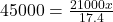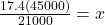## A 45000 watt crane operating at full power lifts a 2100 kg object vertically for 17.4 seconds. How high has the crane lifted the object?

Question

A 45000 watt crane operating at full power lifts a 2100 kg object vertically for 17.4 seconds. How high has the crane lifted the object?

in progress 0
6 months 2021-07-26T12:46:41+00:00 1 Answers 5 views 0

## Answers ( )

Explanation:

We need the power equation here, which is:

Power = (F * Δx)/time

where F * Δx is the amount of work done.

F is a force which is measured in Newtons. We are given the mass of the object, but since we need a Force measure, we need the weight of the object:

F = 2100(9.0)

F = 21000 to the correct number of sig dig.

Now we can plug in the values we have and solve for the displacement, Δx:and isolating x:so

x = 37 m